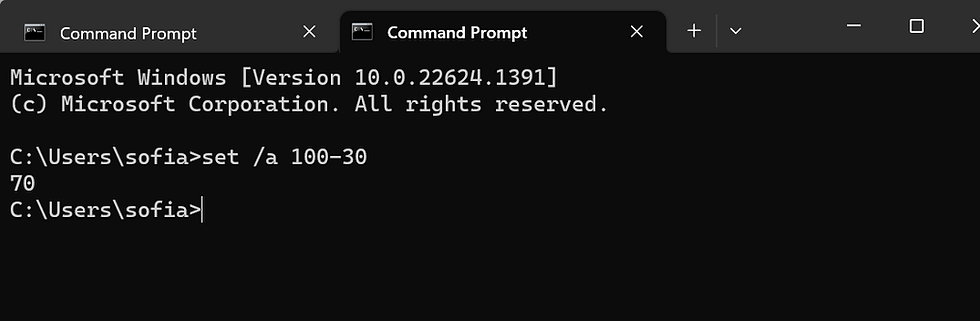top of page
Search

# Perform Arithmetic operations in Command Prompt on Windows 10

Updated: Mar 22

The Command Prompt in Windows 10 is a powerful tool that can be used to perform a variety of tasks. One of the tasks that you can perform with the Command Prompt is arithmetic operations. In this article, we will discuss how to perform arithmetic operations in Command Prompt on Windows 10.

## Perform Arithmetic operations in Command Prompt

First, open the Command Prompt by typing "cmd" in the search bar and clicking on the Command Prompt app.he command to do addition for two or more numbers is:

`set /a num1+num2+num3`

Replace num1, num2, etc., with actual numbers (like 5, 10, or something else) and press Enter. This will show the result.

Alternatively, you can execute the addition command like:

`set /a result= num1+num2+num3`

Again, replace num1, num2 values with actual numbers and it will show the addition result.

### 3] SubtractionThe subtraction command is also very simple. You can perform it for two or more numbers. Here it is:

`set /a num1-num2-num3`

Add actual numbers and the result will be in front of you.

In addition to that, you can also perform subtraction with addition, multiplication, and/or division operations in one go. The command would be:

`set /a num1+num2-num3*num4/num6`

### 2] DivisionFor division operation, the command is:

`set /a num1/num2`

You can also perform multiplication and division operations together. The command would be:

`set /a result=num1*num2/num3`

Execute the command and it will provide the output.

### 4] MultiplicationTo perform multiplication using Command Prompt, the command is:

`set /a num1*num2*num3`

Alternatively, you can also execute this command like this:

`set /a result= num1*num2`

Multiplication can also be done with addition, subtraction, and division together. Just add numbers accordingly and get the result.

### Conclusion

Performing arithmetic operations in Command Prompt on Windows 10 is a simple and efficient way to do quick calculations without the need for a calculator. By using the appropriate symbols for addition, subtraction, multiplication, division, and modulus, as well as following the order of operations, you can easily perform complex calculations.